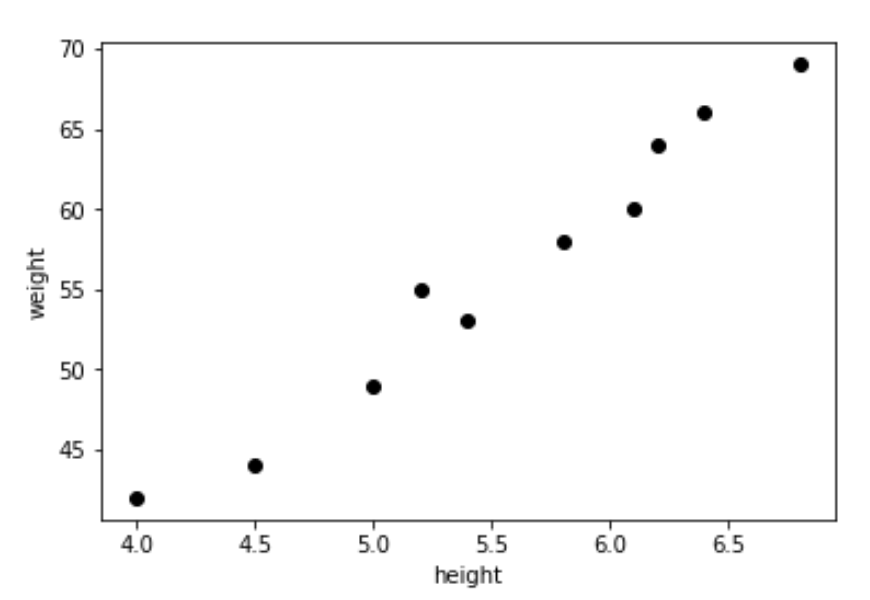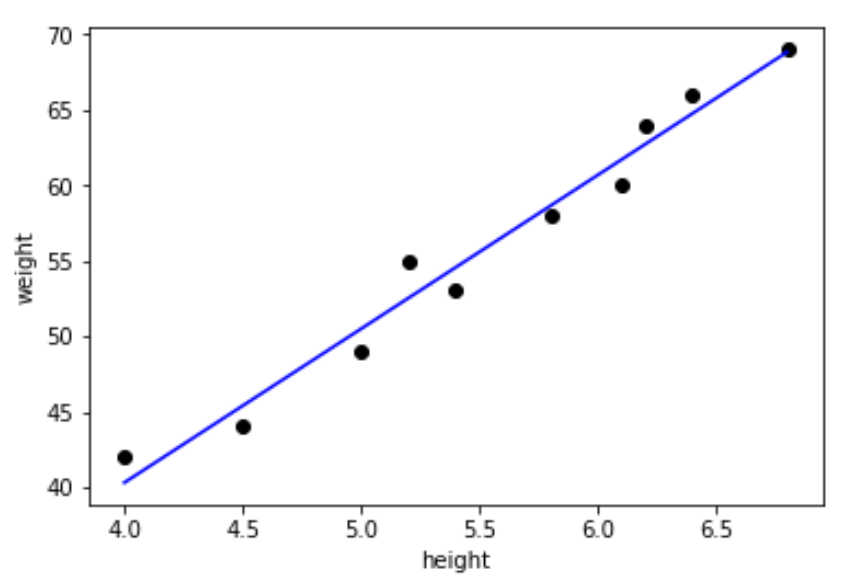# Linear Regression Using Python scikit-learn

### Let's say you have some people's height and weight data. Can you use it to predict other people's weight? Find out using Python scikit-learn.

· AI Zone · Tutorial
Save
21.63K Views

In this article, I am going to explain how to use scikit-learn/sk-learn, a machine learning package in Python, to do linear regression for a set of data points.

Below is a video tutorial on this:

I am not going to explain training data, testing data, and model evaluation concepts here, but they are important.

We know that the equation of a line is given by y=mx+b, where m is the slope and b is the intercept.

Our goal is to find the best values of slope (m) and intercept (b) to fit our data.

Linear regression uses the ordinary least squares method to fit our data points.

`import` statement:

``from sklearn import linear_model``

I have the height and weight data of some people. Let's use this data to do linear regression and try to predict the weight of other people.

``````height=[[4.0],[4.5],[5.0],[5.2],[5.4],[5.8],[6.1],[6.2],[6.4],[6.8]]
weight=[  42 ,  44 , 49, 55  , 53  , 58   , 60  , 64  ,  66 ,  69]

print("height weight")
for row in zip(height, weight):
print(row,"->",row)``````

Output:

``````height weight
4.0 -> 42
4.5 -> 44
5.0 -> 49
5.2 -> 55
5.4 -> 53
5.8 -> 58
6.1 -> 60
6.2 -> 64
6.4 -> 66
6.8 -> 69``````

`import` statement to plot graph using `matplotlib`:

``import matplotlib.pyplot as plt``

Plotting the height and weight data:

``````plt.scatter(height,weight,color='black')
plt.xlabel("height")
plt.ylabel("weight")``````

Output:Declaring the linear regression function and call the `fit` method to learn from data:

``````reg=linear_model.LinearRegression()
reg.fit(height,weight)``````

Slope and intercept:

``````m=reg.coef_
b=reg.intercept_
print("slope=",m, "intercept=",b)``````

Output:

``slope= 10.1936218679 intercept= -0.4726651480``
Using the values of slope and intercept to construct the line to fit our data points:
``````plt.scatter(height,weight,color='black')
predicted_values = [reg.coef_ * i + reg.intercept_ for i in height]
plt.plot(height, predicted_values, 'b')
plt.xlabel("height")
plt.ylabel("weight")``````
Output:And that's it!

#### Linear regression in python scikit learn | Quick KT

Topics:
machine learning, linear regression, python, scikit-learn, sklearn, ai, predictive analytics, tutorial

Published at DZone with permission of Vinay Kumar.

Opinions expressed by DZone contributors are their own.

Comments